• 子类：Son类
• 父类：Father类

## 5个模块：

（1）：直接调用父类属性方法

（2）：重写父类属性方法

（3）：强制调用父类私有属性方法

（4）：调用父类的__init__方法

（5）：继承父类初始化过程中的参数

## （1）调用父类属性方法

``class Father():    def __init__(self):        self.a='aaa'      def action(self):        print('调用父类的方法') class Son(Father):    pass son=Son()     # 子类Son 继承父类Father的所有属性和方法son.action()  # 调用父类方法son.a         # 调用父类属性``## （2）重写父类属性方法

``class Father():    def __init__(self):        self.a='aaa'        def action(self):        print('调用父类的方法') class Son(Father):    def __init__(self):        self.a='bbb'    def action(self):        print('子类重写父类的方法') son=Son()     # 子类Son继承父类Father的所有属性和方法son.action()  # 子类Son调用自身的action方法而不是父类的action方法son.a``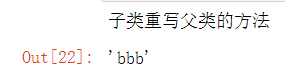``#如果在重新父类方法后，调用父类的方法class Father():    def action(self):        print('调用父类的方法') class Son(Father):    def action(self):        super().action()son=Son()son.action() ``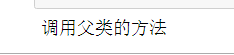## （3）强制调用父类私有属性方法

``class Father():    def __action(self):  # 父类的私有方法        print('调用父类的方法') class Son(Father):    def action(self):        super()._Father__action()son=Son()son.action()``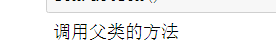## （4）调用父类的__init__方法

``class Father():    def __init__(self):        self.a=a class Son(Father):    def __init__(self):        pass    son=Son()print(son.a)``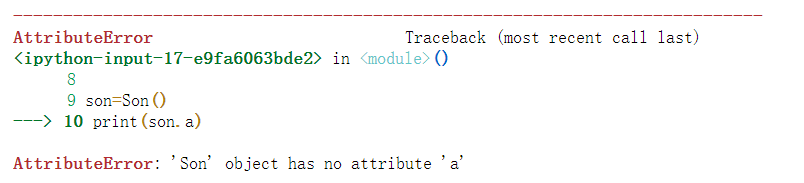``class Father():    def __init__(self):        self.a='aaa' class Son(Father):    def __init__(self):        super().__init__()        #也可以用 Father.__init__(self)  这里面的self一定要加上son=Son()print(son.a) ``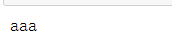## （5）继承父类初始化过程中的参数

``class Father():    def __init__(self):        self.a=1        self.b=2 class Son(Father):    def __init__(self):        super().__init__()        #也可以用 Father.__init__(self)  这里面的self一定要加上    def add(self):        return self.a+self.bson=Son()print(son.add())``

输出结果：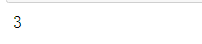1）：参数c为初始化默认参数，如下面的5.1代码中Son(1,2)，参数1,2分别表示a,b的值，默认为c=10，即等于：Son(1,2,10)，表明初始化传参过程中c可以不写

2）：显式地将初始化参数c修改为其他值，如下面的5.2代码中Son(1,2,1)，参数值1,2,1分别表示a,b,c的值，即显式地将c=1传入

``class Father():    def __init__(self,a,b):        self.a = a        self.b = b    def dev(self):        return self.a - self.b  #调用父类初始化参数a,b并增加额外参数cclass Son(Father):    def __init__(self,a,b,c=10):  # 固定值： 例如默认c=10，也可以显示地将c赋值        Father.__init__(self,a,b)          self.c = c    def add(self):        return self.a+self.b    def compare(self):        if self.c > (self.a+self.b):            return True        else:            return False        son=Son(1,2)         # 由于c在初始化过程中默认为10，所以c可以不用显示表达出来print(son.dev())     # 调用父类dev函数print(son.add())     # 子类自身add函数print(son.compare()) # 子类自身compare函数``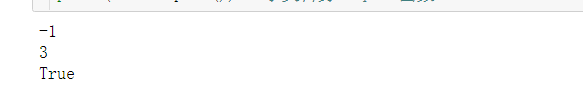``class Father():    def __init__(self,a,b):        self.a = a        self.b = b    def dev(self):        return self.a - self.b  #调用父类初始化参数a,b并增加额外参数cclass Son(Father):    def __init__(self,a,b,c=10):  # 固定值： 例如默认c=10，也可以显式地将c赋值        Father.__init__(self,a,b)          self.c = c    def add(self):        return self.a+self.b    def compare(self):        if self.c > (self.a+self.b):            return True        else:            return False        son=Son(1,2,1)     #  显式地将c=1传入子类初始化函数print(son.dev())     # 调用父类dev函数print(son.add())     # 子类自身add函数print(son.compare()) # 子类自身compare函数``（1）Python类的实例化，封装，继承，私有变量和私有方法

（2）Python-实例方法静态方法类方法对比总结